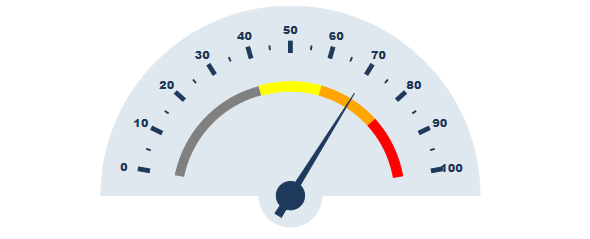﻿ HowTo: Create Half-Circle WijRadialGauge using AngularJS# HowTo: Create Half-Circle WijRadialGauge using AngularJS

The Wijmo demo sample depicts how you can create half circle WijRadialGauge using standard JQuery syntax. This simple blog describes how you can create half circle WijRadialGauge using AngularJS. It clarifies the syntax for the various WijRadialGauge options. The final output is similar to the demo sample. Here is the AngularJS syntax to create half circle WijRadialGauge:

``````<wij-radialgauge value="70" max="100" min="0" radius="190" start-angle="10" sweep-angle="160">
<ranges>
<range start-value="0" end-value="40" start-distance="0.6" end-distance="0.58" start-width="10" end-width="10" >
<style fill="Grey" stroke="none"></style>
</range>
<range start-value="40" end-value="60a" start-distance="0.58" end-distance="0.58" start-width="10" end-width="10">
<style fill="Yellow" stroke="Yellow" stroke-width="1.5"></style>
</range>
<range start-value="60" end-value="80" start-distance="0.58" end-distance="0.58" start-width="10" end-width="10">
<style fill="Orange" stroke="Orange" stroke-width="1.5"></style>
</range>
<range start-value="80" end-value="100" start-distance="0.58" end-distance="0.58" start-width="10" end-width="10">
<style fill="Red" stroke="Red" stroke-width="1.5"></style>
</range>
</ranges>
<face template="face"></face>
Here is the final output:Download the complete sample for detailed implementation.